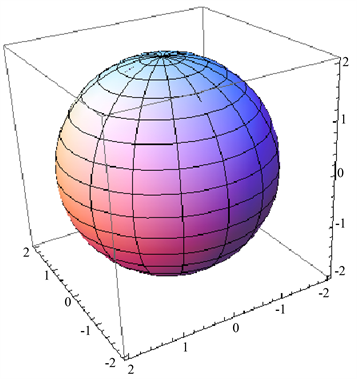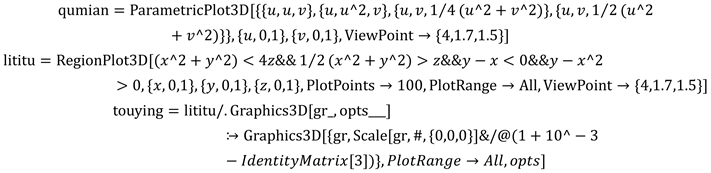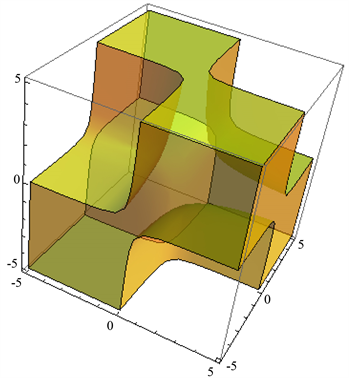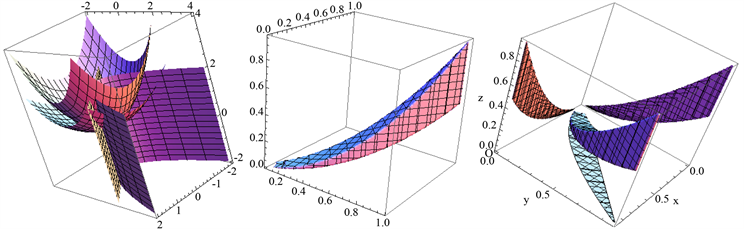# 用Mathematica软件求解三维图形投影的边界线方程Solving the Boundary Equation of 3D Projection with Mathematica

• 全文下载: PDF(1944KB)    PP.323-327   DOI: 10.12677/AAM.2018.74039
• 下载量: 802  浏览量: 3,420

In the case of a given surface equation, we need to obtain the equation of the boundary line of the projection of multiple surfaces in three coordinate planes. We can use Mathematica to draw space graphics to visualize the problem. Through the Mathematica software function of visualization technology and choice of options, we can obtain the ideal space graph, easy to solve the boundary equation problem and solve other related projection problems, such as the simplification of the integral solution process.

1. 引言

2. Mathematica绘制空间图形的基本方法

Mathematica软件中绘制空间曲面的基本函数为Plot3D，命令Plot3D主要用于绘制二元函数z = f(x, y)的图形，该命令的基本格式为Plot3D [f[x, y], {x, x1, x2}, {y, y1, y2}, 选项]。其中f[x, y]是x，y的二元函数，x1，x2表示x的作图范围，y1，y2表示y的作图范围。Plot3D有许多选项，其中常用的如PlotPoints和ViewPoint。PlotPoints的默认值为15，可以增加一些点以使曲面更加精致。选项ViewPoint用于选择图形的视点，默认值为ViewPoint- > {1.3, −2.4, 2.0}。

3. 曲面方程的参数化Figure 1. Paraboloid of revolutionFigure 2. Sphere

RegionPlot3D [xyz < 1, {x, −5, 5}, {y, −5, 5}, {z, −5, 5}, PlotStyle → Directive [Yellow, Opacity [0.5]], Mesh → None]。

4. 确定投影方程

4.1. 演示空间曲面及其投影的生成过程4.2. 确定投影边界线方程并积分Figure 3. Region border diagramFigure 4. Three-dimensional projection drawing process

XOY面： $y=x,y={x}^{2};$

XOZ面： $z=\frac{1}{4}{x}^{2},x=0,z=\frac{1}{2}{x}^{2};$

YOZ面： $z=\frac{1}{4}{y}^{2},y=0,z=\frac{1}{2}{y}^{2}.$

$\begin{array}{c}\underset{D}{\iint }f\left(x,y\right)\text{d}x\text{d}y=\underset{D}{\iint }\left(\frac{\partial Q}{\partial x}-\frac{\partial P}{\partial y}\right)\text{d}x\text{d}y={\int }_{\partial D}P\text{d}x+Q\text{d}y\\ =\underset{a}{\overset{b}{\int }}\text{d}x\underset{y1\left(x\right)}{\overset{y2\left(x\right)}{\int }}f\left(x,y\right)\text{d}y=\underset{a}{\overset{b}{\int }}\text{d}y\underset{x1\left(y\right)}{\overset{x2\left(y\right)}{\int }}f\left(x,y\right)\text{d}x\end{array}$

5. 总结

  丁大正. 科学计算强楷Mathematica 4教程[M]. 北京: 电子工业出版社, 2002.  [美] D.尤金. Mathematica使用指南[M]. 邓建松, 彭冉冉, 译. 北京: 科学出版社, 2002.  王高峡. 用Mathematica软件绘制空间图形的方法和技巧[J]. 重庆工学院学报(自然科学版), 2007, 21(7): 17-20.